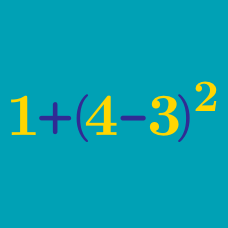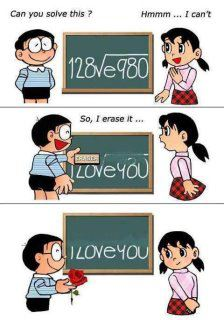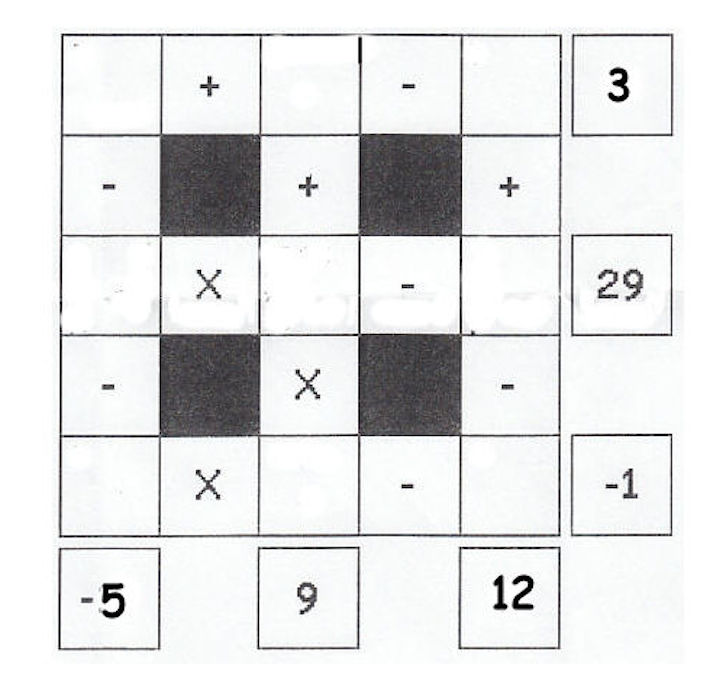Basic Mathematics

# Basic Arithmetic: Level 2 Challenges

$\large \color{#D61F06}3^{\color{#3D99F6}{1506}} - \color{#D61F06}3^{\color{#3D99F6}{1505}} = \ ?$As the photo above shows, the following equation:

$128\sqrt{e980}$

gives the message "I Love You" when half of it is erased. However, what is the value of $128\sqrt{e980}$ when rounded down to one decimal place?

Photo credits: iblogangela

Details and assumptions: $e980$ is defined as $e\times980$.

Please try not to use a calculator for this.

Evaluate $\left( 20142014 \times 20142014-20142004 \times 20142024 \right).$

$\Large \color{#3D99F6}{n}^{\color{#D61F06}{200}} < \color{#20A900}{5}^{\color{#624F41}{300}}$

Find the largest integer $n$ that satisfies the above inequality.Fill the empty squares with integers from 1 to 9, using each integer exactly once, to get the given results. Calculations are done from left to right, and from top to bottom.

What is the sum of the sums of numbers on the 2 diagonals?

Note that the center square is added twice.

×

Problem Loading...

Note Loading...

Set Loading...